Courses

# 28 Year NEET Questions: Chemical Bonding And Molecular Structure- 2

## 30 Questions MCQ Test Chemistry 31 Years NEET Chapterwise Solved Papers | 28 Year NEET Questions: Chemical Bonding And Molecular Structure- 2

Description
This mock test of 28 Year NEET Questions: Chemical Bonding And Molecular Structure- 2 for NEET helps you for every NEET entrance exam. This contains 30 Multiple Choice Questions for NEET 28 Year NEET Questions: Chemical Bonding And Molecular Structure- 2 (mcq) to study with solutions a complete question bank. The solved questions answers in this 28 Year NEET Questions: Chemical Bonding And Molecular Structure- 2 quiz give you a good mix of easy questions and tough questions. NEET students definitely take this 28 Year NEET Questions: Chemical Bonding And Molecular Structure- 2 exercise for a better result in the exam. You can find other 28 Year NEET Questions: Chemical Bonding And Molecular Structure- 2 extra questions, long questions & short questions for NEET on EduRev as well by searching above.
QUESTION: 1

### The correct order of the O–O bond length in O2, H2O2 and O3 is [1995, 2005]

Solution:

The bond length of  O – O in O2 is 1.21 Å, in H2O2 it is 1.48 Å and in O3 it is 1.28 Å.
∴ correct order of bond length is H2O2 > O3 > O2.

QUESTION: 2

### The ground state electronic configuration of valence shell electrons in nitrogen molecule (N2) is written as KK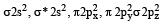Bond order in nitrogen molecule is 

Solution:

In this configuration, there are four completely filled bonding molecular orbitals and one completely filled antibonding molecular orbital. So that Nb = 8 and
Na = 2 .

∴ Bond order =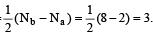QUESTION: 3

### The correct order of N–O bond lengths in NO, NO2–, NO3– and N2O4 is 

Solution:

The N–O bond length decreases in the order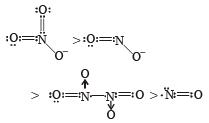QUESTION: 4

Which of the following compounds has a 3-centre bond?

Solution: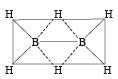The bond represented by dots form the 3-centred electron pair bond. The idea of three centred electron pair bond B–H–B bridges is necessitated because diborane does not have sufficient electrons to  form normal covalent bonds. It has only 12 electrons instead of 14 required to give simple ethane like structure for diborane.

QUESTION: 5

The low density of ice compared to water is due to

Solution:

We know that due to polar nature, water molecules are held together by intermolecular hydrogen bonds. The structure of ice is open with large number of vacant spaces, therfore the density of ice is less than water.

QUESTION: 6

The cylindrical shape of an alkyne is due to the fact that it has 

Solution:

In alkynes the hybridisation is sp i.e each carbon atom undergoes sp hybridisation to form two sp-hybrid orbitals. The two 2porbitals remain unhybridised. Hybrid orbitals form one sigma and two unhybridised orbitals form π-bonds.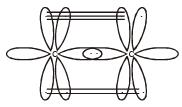Hence two π bond and one sigma bond between C — C lead to cylindrical shape.

QUESTION: 7

N2 and O2 are converted into monocations, N2+ and O2+ respectively. Which of the following statements is wrong ? 

Solution:

For N2;
σ1s2 < σ*1s2 < σ2s2 < σ*2s2 < П2px = 2py4 <σ2pz2 < П*2px = 2py < σ*2pz
For O2;
σ1s2  < σ*1s2 < σ2s2 < σ*2s2 <σ2pz2 < П2px = 2py4  <П*2px = 2py2 < σ*2pz
a) In N2+,the electron is removed from bonding molecular orbital.Hence bond order decreases and  N-N bond weakens.
b) In O2+ , the electron is removed from anti bonding molecular orbital.Hence O-O bond order increases.
c) In O2+, the no of unpaired electrons decreases and hence paramagnetism decreases.
d) In N2+, there is one unpaired electron.It is paramagnetic. This statement is wrong.

QUESTION: 8

The AsF5 molecule is trigonal bipyramidal. The hybrid orbitals used by the As atom for bonding are          

Solution:

The electronic configuration of As is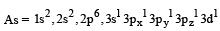sp3 d hybridisation

Thus the hybridisation involved in the AsF5 molecule is trigonal bipyramidal. So, the hybrid orbitals used are s, px, py, pz, dz2.

QUESTION: 9

The number of anti-bonding electron pairs in O2-2 molecular ion on the basis of molecular orbital theory is, (Atomic number of O is 8) 

Solution:

Total no. of electrons in O22- = 16 + 2 = 18

Distribution of electrons in molecular orbital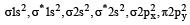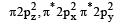Anti bonding electron = 8 (4 pairs)

QUESTION: 10

Among the following which one is not paramagnetic? [Atomic numbers : Be = 4, Ne = 10, As = 33, Cl = 17] 

Solution:

Paramagnetic character is based upon presence of unpaired electron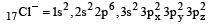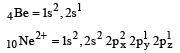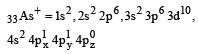Hence only Cl do not have unpaired electrons.

QUESTION: 11

In PO43– ion, the formal charge on each oxygen atom and P—O bond order respectively are

Solution:

Bond order between P – O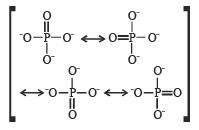or Formal charge on oxygen =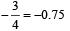(Formal charge = No. of electrons in valance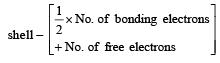QUESTION: 12

Which of the following molecules is planar?

Solution:

XeF4 hybridisation is =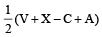hence V = 8 (no. of valence e) X = 4 (no of monovalent atom)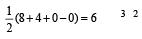C = 0 charge on cation A = 0 (charge on anion). The shape is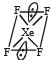Square planar   shape.

QUESTION: 13

The dipole moments of diatomic molecules AB and CD are 10.41D and 10.27 D, respectively while their bond distances are 2.82 and 2.67 Å, respectively. This  indicates that 

Solution:

As dipole moment = electric charge × bond length D. M. of AB molecules = 4.8 x 10 -10 x 2.82 x10 -8 = 13.53D
D.M. of CD molecules = 4.8 x10-10 x 2.67 x10-10 = 12.81D
now% ionic character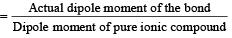then % ionic character  in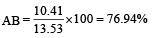% ionic character in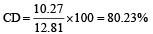QUESTION: 14

Which one of the following arrangements represents the increasing bond orders of the given species? 

Solution: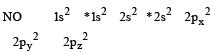Bond order of NO+ =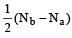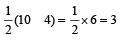Similarly, Bond order of NO =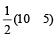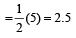Bond order of NO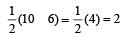Bon d order of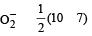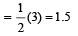By above calculation, we get Decreasing bond order NO+ > NO > NO > O-2

QUESTION: 15

Which one of the following has the pyramidal shape?

Solution:

PF3 has pyramidal shape
Phosphorus exist in sp3 hybridiation state hence it exist in tetrahedral shape. But due to presence of lone pair its shape is pyramidal.
F—H-----F—H-----F—H-----F

QUESTION: 16

Which one of the following molecules will form a linear polymeric structure due to hydrogen bonding? 

Solution:

HF form linear polymer structure due to hydrogen bonding.

QUESTION: 17

Among the following  the electron deficient compound is : 

Solution:

Boron Chloride is the electron deficient compound. B Cl₃.  Boron halides BF₃, BCl₃ and B Br₃ all of them react with Lewis acids to form adducts. The reaction gives out a lot of heat. This tells that the compounds have affinity to electrons and so they react.
Electron deficient compounds have less valence electrons for the atoms in the structure. They have strongly   polarised (electro negative) bonds.
The planar structure of Boron halide is readily converted in to the pyramidal structure. The nature of Pπ bonds give that property to Boron halides. These Boron halides have nature of Lewis acids.
They form adducts with dimethyl sulfide:  BF3 . S(Me)2.  Also with Caesium Chloride:
Cs Cl + B Cl3 => Cs B Cl4

QUESTION: 18

The relationship between the dissociation energy of N2 and N2+ is : 

Solution:

Dissociation energy of any molecules depends upon bond order. Bond order in N2 molecule is 3 while bond order in N+2 is 2.5.
Further we know that more the Bond order, more is the stability and more is the BDE.

QUESTION: 19

Cation and anion combines in a crystal to form following type of compound. 

Solution:

We know that the electr ostatic force that binds the oppositely charged ions which are formed by transfer of electron from one atom to another is called ionic bond. We also know that cation and anion are oppositely charged particles therefore they form ionic bond in crystal.

QUESTION: 20

Among the following ions the pπ–dπ overlap could be present in 

Solution:

In P–O bond, π bond is formed by the sidewise overlapping of d-orbital of P and p-orbital of oxygen. Hence it is formed by pπ and dπ overlapping.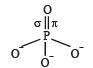QUESTION: 21

In which of the following the bond angle is maximum? 

Solution:

We know that bond angles of  NH3 = 107º, 4+ = 109.5º,  PCl3 = 100º. Therefore bond angle of NH4+ is maximum.

QUESTION: 22

Which of the following two are isostructural?

Solution:

In XeF2 and IF2. Both XeF2 and IF2 are sp3d hybridized and have planar shape.

QUESTION: 23

Main axis of a diatomic molecule is z, molecular orbital px and py overlap to form which of the following orbital? 

Solution:

For π-overlap the lobes of the atomic orbitals are perpendicular to the line joining the nuclei.

QUESTION: 24

In X — H --- Y, X and Y both are electronegative elements

Solution:

In X — H - - - Y, X an d Y both are electronegative elements (i.e attracts the electron pair) then electron density on X will increase and on H will decrease.

QUESTION: 25

Which of the following has pπ – dπ bonding?

Solution:

In SO3-2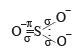S is sp3 hybridised, so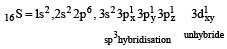In 'S' unhybride d- orbit is present, which is involved in π bond formation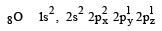n oxygen two unpaired p- orbital is present in these one is involved in σ bond formation while other is used in π bond formation Thus in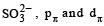orbitals ar e involved for pπ - dπbonding.

QUESTION: 26

In NO3 ion number of bond pair and lone pair of electrons on nitrogen atom respectively are

Solution: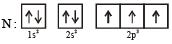To form NO3- , nitrogen uses one p-electron for π-bond formation two p-electrons for σ-bond formation. 2s electrons are used for coordinate bond formation. Thus there is no lone pair on nitrogen but four bond pairs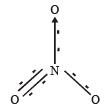QUESTION: 27

Which of the following statements is not correct for sigma and pi-bonds formed between two carbon atoms? 

Solution:

As sigma bond is stronger than the π(pi) bond, so it must be having higher bond energy than π (pi) bond.

QUESTION: 28

Among the following the pair in which the two species are not isostructural is 

Solution:

Shape (structure ) of a species can be ascertained on the basis of hybridisation of its central atom, which in turn can be known by determining the number of hybrid bonds

(H). Thus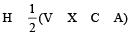For SiF4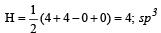(Tetrahedral structure)

For SiF4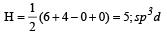(See saw structure)

Hence the two compounds have different structures

QUESTION: 29

In a regular octahedral molecule, MX6 the number of X - M - X bonds at 180° is 

Solution: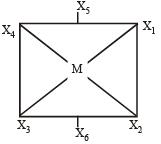Thus here  bond angles between X4 - M - X2 = 180°
X1 - M - X3 = 180°
X5 - M - X6 = 180°

QUESTION: 30

H2O is polar, whereas BeF2 is not. It is because 

Solution:

In a linear symmetrical molecule like BeF2, the bond angle between three atoms is 180°, hence the polarity due to one bond is cancelled by the equal polarity due to other bond, while it is not so in angular molecules, like H2O